Updating search results...

# 42 Results

View
Selected filters:
• Measurement and Data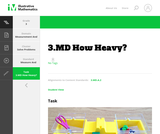Unrestricted Use
CC BY
Rating
0.0 stars

This is a task from the Illustrative Mathematics website that is one part of a complete illustration of the standard to which it is aligned. Each task has at least one solution and some commentary that addresses important aspects of the task and its potential use.

Subject:
Measurement and Data
Material Type:
Activity/Lab
Provider:
Illustrative Mathematics
Provider Set:
Illustrative Mathematics
Author:
Illustrative Mathematics
Date Added:
08/06/2015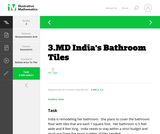Unrestricted Use
CC BY
Rating
0.0 stars

This is a task from the Illustrative Mathematics website that is one part of a complete illustration of the standard to which it is aligned. Each task has at least one solution and some commentary that addresses important aspects of the task and its potential use.

Subject:
Measurement and Data
Material Type:
Activity/Lab
Provider:
Illustrative Mathematics
Provider Set:
Illustrative Mathematics
Author:
Illustrative Mathematics
Date Added:
08/06/2015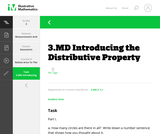Unrestricted Use
CC BY
Rating
0.0 stars

This is a task from the Illustrative Mathematics website that is one part of a complete illustration of the standard to which it is aligned. Each task has at least one solution and some commentary that addresses important aspects of the task and its potential use.

Subject:
Measurement and Data
Material Type:
Activity/Lab
Provider:
Illustrative Mathematics
Provider Set:
Illustrative Mathematics
Author:
Illustrative Mathematics
Date Added:
08/06/2015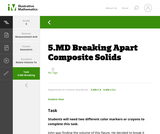Unrestricted Use
CC BY
Rating
0.0 stars

This is a task from the Illustrative Mathematics website that is one part of a complete illustration of the standard to which it is aligned. Each task has at least one solution and some commentary that addresses important aspects of the task and its potential use.

Subject:
Measurement and Data
Material Type:
Activity/Lab
Provider:
Illustrative Mathematics
Provider Set:
Illustrative Mathematics
Author:
Illustrative Mathematics
Date Added:
08/06/2015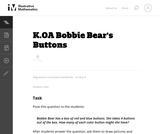Unrestricted Use
CC BY
Rating
0.0 stars

The purpose of this task is for students to find different pairs of numbers that sum to 4.

Subject:
Mathematics
Measurement and Data
Material Type:
Activity/Lab
Provider:
Illustrative Mathematics
Provider Set:
Illustrative Mathematics
Author:
Illustrative Mathematics
Date Added:
05/01/2012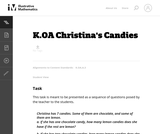Unrestricted Use
CC BY
Rating
0.0 stars

The purpose of this task is for students to find different pairs of numbers that sum to 7.

Subject:
Mathematics
Measurement and Data
Material Type:
Activity/Lab
Provider:
Illustrative Mathematics
Provider Set:
Illustrative Mathematics
Author:
Illustrative Mathematics
Date Added:
05/01/2012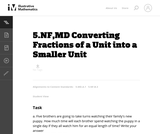Unrestricted Use
CC BY
Rating
0.0 stars

This task gives students word problems with a given a set of a specified size and a specified number of subsets. The questions ask the student to find out the size of each of the subsets.

Subject:
Mathematics
Measurement and Data
Numbers and Operations
Material Type:
Activity/Lab
Provider:
Illustrative Mathematics
Provider Set:
Illustrative Mathematics
Author:
Illustrative Mathematics
Date Added:
05/01/2012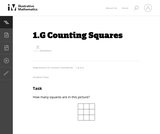Unrestricted Use
CC BY
Rating
3.0 stars

This challenging problem and brainteaser gives first graders an opportunity to compose and decompose squares.

Subject:
Mathematics
Geometry
Measurement and Data
Material Type:
Activity/Lab
Provider:
Illustrative Mathematics
Provider Set:
Illustrative Mathematics
Author:
Illustrative Mathematics
Date Added:
09/02/2012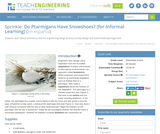Read the Fine Print
Educational Use
Rating
0.0 stars

Students learn about biomimicry and the engineering design process as they design and build model ptarmigan feet.

Subject:
Life Science
Mathematics
Measurement and Data
Material Type:
Activity/Lab
Provider:
TeachEngineering
Provider Set:
TeachEngineering
Date Added:
02/17/2017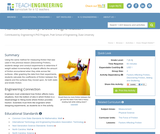Read the Fine Print
Educational Use
Rating
0.0 stars

Using the same method for measuring friction that was used in the previous lesson (Discovering Friction), students design and conduct an experiment to determine if weight added incrementally to an object affects the amount of friction encountered when it slides across a flat surface. After graphing the data from their experiments, students can calculate the coefficients of friction between the object and the surface it moved upon, for both static and kinetic friction.

Subject:
Engineering
Measurement and Data
Material Type:
Activity/Lab
Lesson Plan
Provider:
TeachEngineering
Provider Set:
TeachEngineering
Author:
Mary R. Hebrank
Date Added:
09/26/2008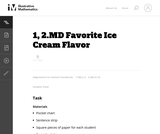Unrestricted Use
CC BY
Rating
4.0 stars

Students have the opportunity to create and interpret data from simple daily questions.

Subject:
Mathematics
Measurement and Data
Material Type:
Activity/Lab
Provider:
Illustrative Mathematics
Provider Set:
Illustrative Mathematics
Author:
Illustrative Mathematics
Date Added:
05/01/2012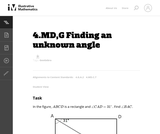Unrestricted Use
CC BY
Rating
3.0 stars

The purpose of this task is to give 4th grade students a problem involving an unknown quantity that has a clear visual representation. Students must understand that the four interior angles of a rectangle are all right angles and that right angles have a measure of 90_ and that angle measure is additive.

Subject:
Mathematics
Geometry
Measurement and Data
Material Type:
Activity/Lab
Provider:
Illustrative Mathematics
Provider Set:
Illustrative Mathematics
Author:
Illustrative Mathematics
Date Added:
10/12/2012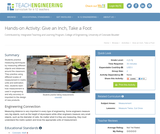Read the Fine Print
Educational Use
Rating
0.0 stars

In this activity students practice measuring techniques by measuring different objects and distances around the classroom. They practice using different scales of measurement in metric units and estimation.

Subject:
Engineering
Measurement and Data
Material Type:
Activity/Lab
Lesson Plan
Provider:
TeachEngineering
Provider Set:
TeachEngineering
Author:
Janet Yowell
Lesley Herrmann
Malinda Schaefer Zarske
Date Added:
09/18/2014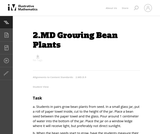Unrestricted Use
CC BY
Rating
0.0 stars

This task adds some rigor to the activity of growing bean plants. By collecting growth data, students practice measuring and recording length measurements.

Subject:
Mathematics
Measurement and Data
Material Type:
Activity/Lab
Provider:
Illustrative Mathematics
Provider Set:
Illustrative Mathematics
Author:
Illustrative Mathematics
Date Added:
05/01/2012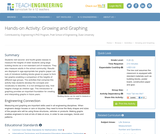Read the Fine Print
Educational Use
Rating
0.0 stars

Students visit second- and fourth-grade classes to measure the heights of older students using large building blocks as a non-standard unit of measure. They also measure adults in the school community. Results are displayed in age-appropriate bar graphs (paper cut-outs of miniature building blocks glued on paper to form bar graphs) enabling a comparison of the heights of different age groups. The activities that comprise this activity help students develop the concepts and vocabulary to describe, in a non-ambiguous way, how heights change as children age. This introduction to graphing provides an important foundation for creating and interpreting graphs in future years.

Subject:
Engineering
Measurement and Data
Material Type:
Activity/Lab
Provider:
TeachEngineering
Provider Set:
TeachEngineering
Author:
Mary R. Hebrank
Date Added:
10/14/2015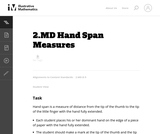Unrestricted Use
CC BY
Rating
3.0 stars

In this activity students measure their hand span. A line graph is used to record the data from the glass.

Subject:
Mathematics
Measurement and Data
Material Type:
Activity/Lab
Provider:
Illustrative Mathematics
Provider Set:
Illustrative Mathematics
Author:
Illustrative Mathematics
Date Added:
05/01/2012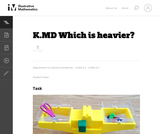Unrestricted Use
CC BY
Rating
0.0 stars

In this activity using a balance scale students practice weighing items to see how heavy they are. Cubes are used in the balance as units of measure so students may easily count them.

Subject:
Mathematics
Measurement and Data
Material Type:
Activity/Lab
Provider:
Illustrative Mathematics
Provider Set:
Illustrative Mathematics
Author:
Illustrative Mathematics
Date Added:
01/11/2013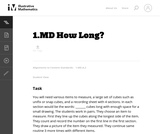Unrestricted Use
CC BY
Rating
3.0 stars

Students work in pairs to measure length by lining up cubes along the longest side of an item. They count and record length by counting the number of cubes.

Subject:
Mathematics
Measurement and Data
Material Type:
Activity/Lab
Provider:
Illustrative Mathematics
Provider Set:
Illustrative Mathematics
Author:
Illustrative Mathematics
Date Added:
06/20/2012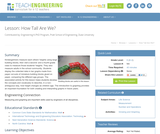Read the Fine Print
Educational Use
Rating
0.0 stars

Kindergartners measure each other's height using large building blocks, then visit a 2nd and a 4th grade class to measure those students. They can also measure adults in the school community. Results are displayed in age-appropriate bar graphs (paper cut-outs of miniature building blocks glued on paper to form a bar graph) comparing the different age groups. The activities that comprise this lesson help students develop the concepts and vocabulary to describe, in a non-ambiguous way, how height changes as children get older. The introduction to graphing provides an important foundation for both creating and interpreting graphs in future years.

Subject:
Engineering
Measurement and Data
Material Type:
Activity/Lab
Lesson Plan
Provider:
TeachEngineering
Provider Set:
TeachEngineering
Author:
Mary R. Hebrank
Date Added:
09/18/2014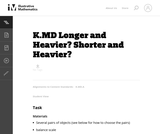Unrestricted Use
CC BY
Rating
0.0 stars

In this activity students compare objects to see which is longer and heavier than the other.

Subject:
Mathematics
Measurement and Data
Material Type:
Activity/Lab
Provider:
Illustrative Mathematics
Provider Set:
Illustrative Mathematics
Author:
Illustrative Mathematics
Date Added:
08/20/2012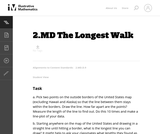Unrestricted Use
CC BY
Rating
0.0 stars

In this task students figure out how to draw the longest line on a map of the United States without hitting a border. They use color and line plots to keep track of their results.

Subject:
Mathematics
Measurement and Data
Material Type:
Activity/Lab
Provider:
Illustrative Mathematics
Provider Set:
Illustrative Mathematics
Author:
Illustrative Mathematics
Date Added:
05/01/2012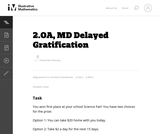Unrestricted Use
CC BY
Rating
5.0 stars

The purpose of this task is for students to compare two options for a prize where the value of one is given \$2 at a time, giving them an opportunity to "work with equal groups of objects to gain foundations for multiplication." This context also provides students with an introduction to the concept of delayed gratification, or resisting an immediate reward and waiting for a later reward, while working with money.

Subject:
Mathematics
Measurement and Data
Numbers and Operations
Material Type:
Activity/Lab
Provider:
Illustrative Mathematics
Provider Set:
Illustrative Mathematics
Author:
Illustrative Mathematics
Date Added:
01/17/2013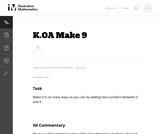Unrestricted Use
CC BY
Rating
3.0 stars

The purpose of this task is for students to find different pairs of numbers that sum to 9.

Subject:
Mathematics
Measurement and Data
Material Type:
Activity/Lab
Provider:
Illustrative Mathematics
Provider Set:
Illustrative Mathematics
Author:
Illustrative Mathematics
Date Added:
05/01/2012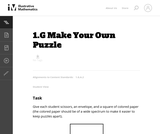Unrestricted Use
CC BY
Rating
0.0 stars

This instructional task gives students a hands-on experience with composing and decomposing geometric figures.

Subject:
Mathematics
Geometry
Measurement and Data
Material Type:
Activity/Lab
Provider:
Illustrative Mathematics
Provider Set:
Illustrative Mathematics
Author:
Illustrative Mathematics
Date Added:
09/09/2012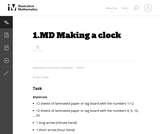Unrestricted Use
CC BY
Rating
3.0 stars

This task is to introduce students to the concept of reading an analog clock.

Subject:
Mathematics
Measurement and Data
Material Type:
Activity/Lab
Provider:
Illustrative Mathematics
Provider Set:
Illustrative Mathematics
Author:
Illustrative Mathematics
Date Added:
10/08/2012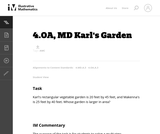Unrestricted Use
CC BY
Rating
3.0 stars

The purpose of the task is for students to solve a multi-step multiplication problem in a context that involves area. In addition, the numbers were chosen to determine if students have a common misconception related to multiplication.

Subject:
Mathematics
Measurement and Data
Numbers and Operations
Material Type:
Activity/Lab
Provider:
Illustrative Mathematics
Provider Set:
Illustrative Mathematics
Author:
Illustrative Mathematics
Institute for Mathematics & Education funded by the Bill & Melinda Gates Foundation
Date Added:
07/24/2012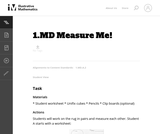Unrestricted Use
CC BY
Rating
0.0 stars

In this task students work with partners to measure themselves by laying multiple copies of a shorter object that represents the length unit end to end. It gives students the opportunity to discuss the need to be careful when measuring.

Subject:
Mathematics
Measurement and Data
Material Type:
Activity/Lab
Provider:
Illustrative Mathematics
Provider Set:
Illustrative Mathematics
Author:
Illustrative Mathematics
Date Added:
01/15/2013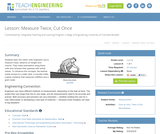Read the Fine Print
Educational Use
Rating
0.0 stars

Students learn the metric units engineers use to measure mass, distance (or length) and volume. They make estimations using these units and compare their guesses with actual values. To introduce the concepts, the teacher needs access to a meter stick, a one-liter bottle, a glass container that measures milliliters and a gram scale.

Subject:
Engineering
Measurement and Data
Material Type:
Lesson Plan
Provider:
TeachEngineering
Provider Set:
TeachEngineering
Author:
Janet Yowell
Lesley Herrmann
Malinda Schaefer Zarske
Date Added:
09/18/2014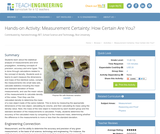Read the Fine Print
Educational Use
Rating
0.0 stars

Students learn about the statistical analysis of measurements and error propagation, reviewing concepts of precision, accuracy and error types. This is done through calculations related to the concept of density. Students work in teams to each measure the dimensions and mass of five identical cubes, compile the measurements into small data sets, calculate statistics including the mean and standard deviation of these measurements, and use the mean values of the measurements to calculate density of the cubes. Then they use this calculated density to determine the mass of a new object made of the same material. This is done by measuring the appropriate dimensions of the new object, calculating its volume, and then calculating its mass using the density value. Next, the mass of the new object is measured by each student group and the standard deviation of the measurements is calculated. Finally, students determine the accuracy of the calculated mass by comparing it to the measured mass, determining whether the difference in the measurements is more or less than the standard deviation.

Subject:
Engineering
Measurement and Data
Material Type:
Activity/Lab
Provider:
TeachEngineering
Provider Set:
TeachEngineering
Author:
Ralph Cox
Date Added:
10/14/2015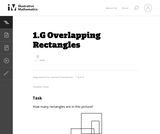Unrestricted Use
CC BY
Rating
4.0 stars

This challenging problem and brainteaser gives students an opportunity to compose and decompose polygons to make rectangles.

Subject:
Mathematics
Geometry
Measurement and Data
Material Type:
Activity/Lab
Provider:
Illustrative Mathematics
Provider Set:
Illustrative Mathematics
Author:
Illustrative Mathematics
Date Added:
08/29/2012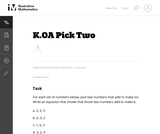Unrestricted Use
CC BY
Rating
3.0 stars

The purpose of this task is for students to select 2 numbers from a set of 3 that sum to 9. The task can be completed for sums equaling any number. Teachers may choose to ask students to write the simple equations they select.

Subject:
Mathematics
Measurement and Data
Material Type:
Activity/Lab
Provider:
Illustrative Mathematics
Provider Set:
Illustrative Mathematics
Author:
Illustrative Mathematics
Date Added:
05/01/2012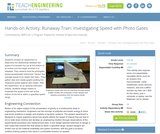Read the Fine Print
Educational Use
Rating
0.0 stars

Students conduct an experiment to determine the relationship between the speed of a wooden toy car at the bottom of an incline and the height at which it is released. They observe how the photogate-based speedometer instrument "clocks" the average speed of an object (the train). They gather data and create graphs plotting the measured speed against start height. After the experiment, as an optional extension activity, students design brakes to moderate the speed of the cart at the bottom of the hill to within a specified speed range.

Subject:
Engineering
Measurement and Data
Material Type:
Activity/Lab
Provider:
TeachEngineering
Provider Set:
TeachEngineering
Author:
Andrew Cave
Date Added:
09/18/2014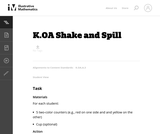Unrestricted Use
CC BY
Rating
3.0 stars

The purpose of this task is for students to select 2 numbers from a set that sum to 5 (or any other number).

Subject:
Mathematics
Measurement and Data
Material Type:
Activity/Lab
Provider:
Illustrative Mathematics
Provider Set:
Illustrative Mathematics
Author:
Illustrative Mathematics
Date Added:
05/01/2012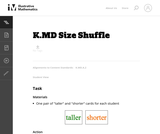Unrestricted Use
CC BY
Rating
0.0 stars

In this activity students become familiar with the words, Ňtaller/shorterÓ as they refer to height.

Subject:
Mathematics
Measurement and Data
Material Type:
Activity/Lab
Provider:
Illustrative Mathematics
Provider Set:
Illustrative Mathematics
Author:
Illustrative Mathematics
Date Added:
05/01/2012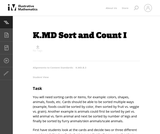Unrestricted Use
CC BY
Rating
0.0 stars

In this activity students become familiar with the math vocabulary more/less/same and most/least as they count and compare small groups.

Subject:
Mathematics
Measurement and Data
Material Type:
Activity/Lab
Provider:
Illustrative Mathematics
Provider Set:
Illustrative Mathematics
Author:
Illustrative Mathematics
Date Added:
06/20/2012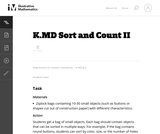Unrestricted Use
CC BY
Rating
0.0 stars

This activity builds on Sort and Count I. It also helps students become familiar with the math vocabulary more/less/same and most/least as they sort, count, and compare small groups of objects.

Subject:
Mathematics
Measurement and Data
Material Type:
Activity/Lab
Provider:
Illustrative Mathematics
Provider Set:
Illustrative Mathematics
Author:
Illustrative Mathematics
Date Added:
08/21/2012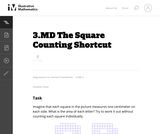Unrestricted Use
CC BY
Rating
0.0 stars

This is a rectangle subdivision task; ideally instead of counting each square. students should break the letters into rectangles, multiply to find the areas, and add up the areas. However, students should not be discouraged from using individual counting to start if they are stuck. Often students will get tired of counting and devise the shortcut method themselves.

Subject:
Mathematics
Measurement and Data
Material Type:
Activity/Lab
Provider:
Illustrative Mathematics
Provider Set:
Illustrative Mathematics
Author:
Illustrative Mathematics
Date Added:
05/01/2012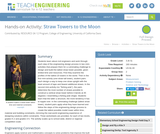Read the Fine Print
Educational Use
Rating
0.0 stars

Students learn about civil engineers and work through each step of the engineering design process in two mini-activities that prepare them for a culminating challenge to design and build the tallest straw tower possible, given limited time and resources. First they examine the profiles of the tallest 20 towers in the world. Then in the first mini-activity (one-straw tall tower), student pairs each design a way to keep one straw upright with the least amount of tape and fewest additional straws. In the second mini-activity (no "fishing pole"), the pairs determine the most number of straws possible to construct a vertical straw tower before it bends at 45 degrees—resembling a fishing pole shape. Students learn that the taller a structure, the more tendency it has to topple over. In the culminating challenge (tallest straw tower), student pairs apply what they have learned and follow the steps of the engineering design process to create the tallest possible model tower within time, material and building constraints, mirroring the real-world engineering experience of designing solutions within constraints. Three worksheets are provided, for each of two levels, grades K-2 and grades 3-5. The activity scales up to school-wide, district or regional competition scale.

Subject:
Mathematics
Measurement and Data
Material Type:
Activity/Lab
Provider:
TeachEngineering
Provider Set:
TeachEngineering
Author:
Tiffany Tu
Date Added:
02/17/2017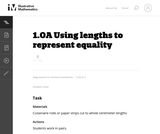Unrestricted Use
CC BY
Rating
4.0 stars

Students develop a physical understanding for the meaning of equality by trying to find equal lengths using rods.

Subject:
Mathematics
Measurement and Data
Numbers and Operations
Material Type:
Activity/Lab
Provider:
Illustrative Mathematics
Provider Set:
Illustrative Mathematics
Author:
Illustrative Mathematics
Date Added:
09/20/2012